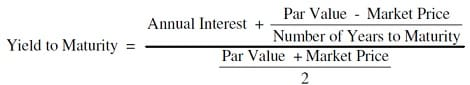Share:

YTM or yield-to-maturity is a term used very closely with bonds. Therefore YTM becomes a relevant concept for debt mutual funds. YTM is expressed as an annual return. It tells us the total return that is expected from a bond if the investor holds the bond until maturity. The underlying assets of a debt fund is a collection of different government and corporate bonds that a fund manager chooses to keep in the portfolio.

Yield to Maturity Meaning

Yield to maturity is the rate of return, mostly annualised, that an investor can expect to earn if they hold the bond till maturity. Same is the case with a fund manager holding bonds in the mutual fund portfolio. YTM assumes that the investor has reinvested all the coupon payments received from the bond back into it until maturity. At times, it also considers the reinvestment of principal amount at maturity.

What is a Coupon?

Coupon payment is the annual rate of interest that is given to a bondholder. The coupon rate is more or less fixed.

## How do YTMs work?

The price at which the bond can be bought from the market will tell you what the present value of all the cash flows in future.

But, bonds are marketable securities, and the prices fluctuate with moving interest rates in the economy.

Now, here’s the catch. The coupon rate of the bond remains fixed until maturity. What fluctuates is the price and the yield. So if a bond with a face value of Rs 2,000 has a coupon rate of 5%. Then, the coupon rate will stay so until maturity. The price may go up or down. When the interest rates in the market rise, more than the coupon rate being offered on the bonds, the bond looks less attractive. This pushes the price of the bond downwards. The opposite happens with the interest rates fall. This makes the bond more attractive as the coupon rates are higher, pushing the bond price upwards.

### Mutual funds for all your goals

•Instant investment

•Zero commission

•Completely paperless

If you look at the yield to maturity formula, you will see that the calculation of YTM assumes that all coupon payments are invested back into the bond so that the present value of cash flows equals the bond’s market price in current times. The rate at which cash flows are assumed to be invested is called YTM of the bond.

## Yield to Maturity Example

Let’s understand how YTM’s work with an example. Suppose a company, ABC Ltd., issues 8% annual bonds of Rs 2,000.

here

Coupon rate: 8%

Periodicity of payment: Once in a year (Annual)

Face value: Rs 2,000

Date of issue: August 17, 2020

Maturity: Five years

If you buy the bond when it is issued, you will be buying the bond at face value which will also be your purchase price. The bonds will pay the coupons at 8% or Rs 160 on August 17, 2021.

If the bond is selling for a lower price than the face value, this means that the going interest rate is higher than the coupon rate. Here YTM will be higher than the coupon rate, which is 8%. If the bond is selling for a higher price than the face value, this means the interest rate in the market is lower than the coupon rate. This indicates that the YTM is lesser than the coupon rate.

## Current Yield

YTM is one of the ways that a bond yield can be represented and is useful to investors. Yield can also be represented in the form of current yield.

Let’s again look at our yield to maturity example to understand what is the current yield.

Current yield, by definition, is the annual rate of return that you receive for the price paid for that bond.

The formula of current yield: Coupon rate / Purchase price

Naturally, if the bond purchase price is equal to the face value, current yield will be equal to the coupon rate.

Current Yield= 160/2,000 = 0.08 or 8%

Let’s say the purchase price falls to 1,800

Current Yield= 160/1,800= 0.089 or 8.9%

Current Yield rises if the purchase price falls.

## YTM Formula

The formula to calculate YTM is:Let’s use this YTM formula for our example.

Assumptions:

Years to maturity (August 17, 2025)= 4

Coupon: 8%

Face/Par value: Rs 2,000

Current Market Price: Rs 1,800

YTM= 11.05%

## Conclusion: How is it useful for you

YTM can be used as a metric to decide if a bond is a good investment or not. It helps to decide if a bond is worth investing or not. You can compare the YTM of a bond with other bonds to see which one has a better road to maturity. YTM can also be used to compare bonds with different maturities for a like to like comparison. The important point to know is that YTM gives you expected returns. Even though bonds are far less volatile than stocks, nothing in the world of investments can be taken by the stone. Make an informed decision and look at other factors as well.

Share: